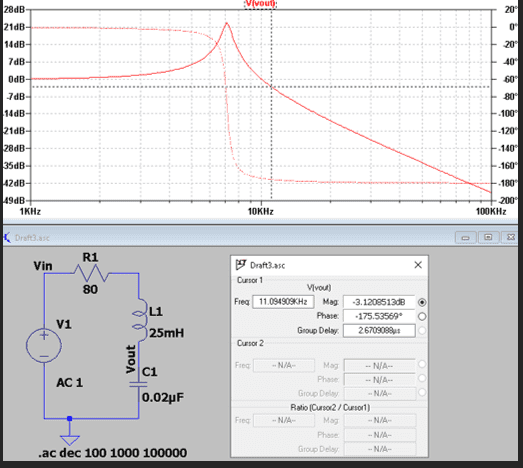# Cutoff frequency for this RLC circuit

• IronaSona
In summary, the cutoff frequency of an RLC circuit can be found using the equation ##f_{\rm c.o.} = \frac{1}{2\pi}\left[\frac{1}{LC} - \frac{R^2}{2L^2}+\sqrt{\frac{2}{L^2C^2}-\frac{R^2}{L^3C}+\frac{R^4}{4L^4}} \right]^{1/2}## which is derived from the voltage divider magnitude being set equal to ##\frac {1}{\sqrt{2}}##. This equation may give a different result than the more commonly known 1/(2*pi*sqrt(L*C

#### IronaSona

Homework Statement
Cut off frequency
Relevant Equations
1/2*pi*L*C
How would i find the cut of frequency of this RLC circuit .I have used LTspice to find it but what is the equation for finding itDid you try a web search for "cutoff frequency LRC circuit"? For example, I found https://www.electronics-tutorials.ws/accircuits/series-resonance.html

You should look for at least one more source on the web in order to check the formulas.

••DaveE and Delta2
It depends how you define the cut off frequency. The article given in the link of post #2 defines the cutoff frequency as the frequency (of the source) that the amplitude of the current in the circuit is equal to 70.7% of its maximum(resonant) value. There are two possible values of reactance ##X=\omega L-\frac{1}{\omega C}## to realize this current , ##X=-R## and ##X=R##. Solving $$-R=\omega_1L-\frac{1}{\omega_1 C}$$ gives the lower cutoff frequency ##\omega_1## and solving $$R=\omega_2L-\frac{1}{\omega_2 C}$$ gives the higher cutoff frequency ##\omega_2##

TSny said:
Did you try a web search for "cutoff frequency LRC circuit"? For example, I found https://www.electronics-tutorials.ws/accircuits/series-resonance.html

You should look for at least one more source on the web in order to check the formulas.
when i search it i get 1/2*pi*L*C formula , and when i use it i get answer or 8kHz but the simulation is showing 11.1kHz

IronaSona said:
when i search it i get 1/2*pi*L*C formula , and when i use it i get answer or 8kHz but the simulation is showing 11.1kHz
##\frac{1}{2\pi\sqrt{LC}}## is the frequency of resonance, not the cutoff frequency. I suggest you read carefully the link of post #2, in post #3 I give a summary of the most important points.

Delta2 said:
It depends how you define the cut off frequency. The article given in the link of post #2 defines the cutoff frequency as the frequency (of the source) that the amplitude of the current in the circuit is equal to 70.7% of its maximum(resonant) value. There are two possible values of reactance ##X=\omega L-\frac{1}{\omega C}## to realize this current , ##X=-R## and ##X=R##. Solving $$-R=\omega_1L-\frac{1}{\omega_1 C}$$ gives the lower cutoff frequency ##\omega_1## and solving $$R=\omega_2L-\frac{1}{\omega_2 C}$$ gives the higher cutoff frequency ##\omega_2##
what is the w is that the 2*pi

IronaSona said:
what is the w is that the 2*pi
##\omega## is the angular frequency is is equal to ##\omega=2\pi f## where ##f## the frequency

Delta2 said:
##\omega## is the angular frequency is is equal to ##\omega=2\pi f## where ##f## the frequency
o ok thank you

•Delta2
I now see that the ##V_{out}## is the capacitor's voltage, this implies a different cut off frequency than the ones I mention in post #3.

•TSny
If you define the cut-off frequency as the -3dB point, then the equation you're looking for is a mess.
It will be the solution(s)* to this equation: ## L^2C^2\omega^4+(R^2C^2-2LC)\omega^2-1=0 ##

I suspect that they are really asking for the resonant frequency, or they expect you to make an approximation based on the extension of the high and low frequency asymptotes. Both of which are much easier to derive.

The other approach would be to put in the values immediately, which makes the algebra easier for that equation. But it isn't very instructive; not much different than asking LTSpice, really.

Ask me if you want to see how I derived that equation, it is really just a voltage divider magnitude (with complex impedances) set equal to ##\frac {1}{\sqrt{2}}##

*there should be only one solution that is physically realizable.

•TSny
As @Delta2 pointed out, the link in post #2 is for the case where you are looking at the output voltage across the resistor instead of the capacitor. So, that link does not give the formula for the cutoff frequency for your situation.

As @DaveE noted in the previous post, finding the -3dB cutoff frequency involves solving the quadratic equation that he wrote down. The solution is a little messy. (I let Mathematica find it!)
$$f_{\rm c.o.} = \frac{1}{2\pi}\left[\frac{1}{LC} - \frac{R^2}{2L^2}+\sqrt{\frac{2}{L^2C^2}-\frac{R^2}{L^3C}+\frac{R^4}{4L^4}} \right]^{1/2}$$ You can plug in your values for ##R, L## and ##C## to see if you get a cutoff frequency of about 11 kHz.

•Delta2 and IronaSona
TSny said:
As @Delta2 pointed out, the link in post #2 is for the case where you are looking at the output voltage across the resistor instead of the capacitor. So, that link does not give the formula for the cutoff frequency for your situation.

As @DaveE noted in the previous post, finding the -3dB cutoff frequency involves solving the quadratic equation that he wrote down. The solution is a little messy. (I let Mathematica find it!)
$$f_{\rm c.o.} = \frac{1}{2\pi}\left[\frac{1}{LC} - \frac{R^2}{2L^2}+\sqrt{\frac{2}{L^2C^2}-\frac{R^2}{L^3C}+\frac{R^4}{4L^4}} \right]^{1/2}$$ You can plug in your values for ##R, L## and ##C## to see if you get a cutoff frequency of about 11 kHz.
thank you , ill try it .

If ##\large \frac R L## is small compared to ##\large \frac{1}{\sqrt{LC}}##, the expression for ##f_{\rm c.o.}## can be approximated as $$f_{\rm c.o} \approx \frac{1}{2 \pi}\left[\frac{1+\sqrt{2}}{LC}\right]^{1/2}= \left(1+\sqrt{2}\right)^{1/2}f_{\rm resonance}$$

This approximation works well for your setup.

Last edited:
•IronaSona and Delta2